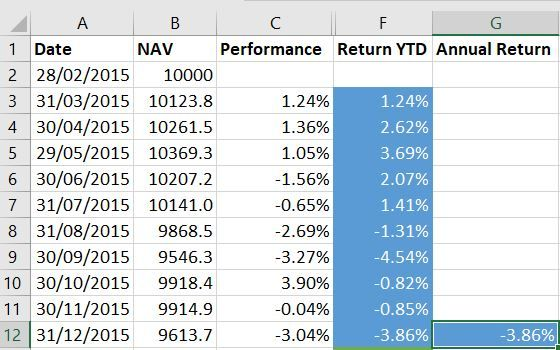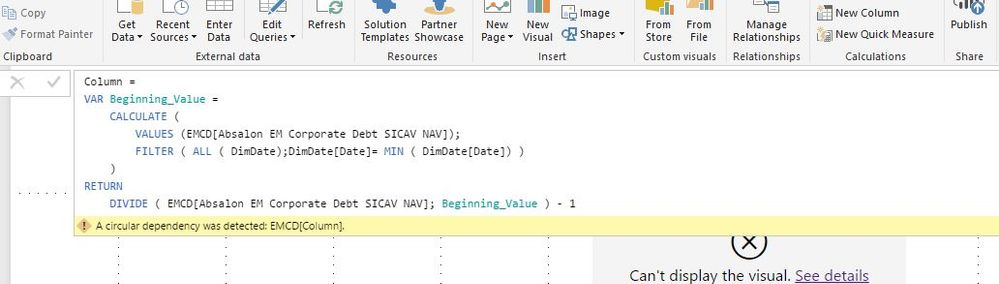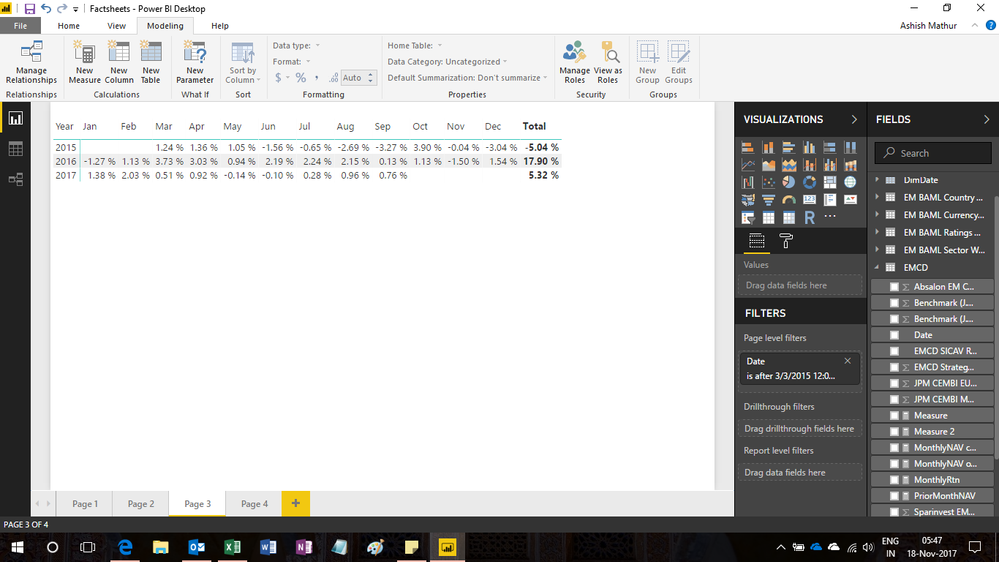cancel
Showing results for
Did you mean:Helper III

## Annual Returns

I am trying to calculate a fund's yearly performance based on monthly returns. In excel we calculate the yearly performance with the formula B12/B2-1 which gives us the total performance for 2015 of -3.86% (see attached photo).  How would we calculate this formula in dax?1 ACCEPTED SOLUTIONCommunity Champion

HOw abou this one?

```YTD_Zubair =
VAR Previous_YEAR_Closing =
CALCULATE (
MAX ( EMCD[Date] ),
EMCD[Date] < EARLIER ( EMCD[Date] )
&& YEAR ( EMCD[Date] )
= YEAR ( EARLIER ( EMCD[Date] ) ) - 1
)
RETURN
DIVIDE (
EMCD[Absalon EM Corporate Debt SICAV NAV],
CALCULATE (
SUM ( EMCD[Absalon EM Corporate Debt SICAV NAV] ),
EMCD[Date] = Previous_YEAR_Closing
)
)
- 1```

Regards
Zubair

16 REPLIES 16Community Champion

Try this calculated Column to get YTD return.

```=
VAR Beginning_Value =
CALCULATE (
VALUES ( Table1[NAV] ),
FILTER ( ALL ( Table1 ), Table1[Date] = MIN ( Table1[Date] ) )
)
RETURN
DIVIDE ( Table1[NAV], Beginning_Value ) - 1```

Regards
ZubairHelper III

Thanks Zubair,

I'm clearly doing something wrong as I get the following error. What is causing the circular dependency? Can you point me in the right direction please?Community Champion

Regards
ZubairHelper IIIHelper III

Sorry the last one didn't seem to work hopefully this does https://1drv.ms/x/s!AiRs1BCuVWhM6EfOsWkw52AbDaq-Community Champion

This is an Excel file.

The problem (i believe) is in your PBIX file (the image you shared) which uses mutliple tables. I was looking for that file.

Regards
ZubairHelper IIICommunity Champion

Please see the file attached here

The earliest month of 2015 is empty so I filtered the year 2015 out

```YTD_Zub =
VAR Previous_Date =
CALCULATE (
MIN ( EMCD[Date] ),
EMCD[Date] < EARLIER ( EMCD[Date] )
&& YEAR ( EMCD[Date] ) = YEAR ( EARLIER ( EMCD[Date] ) )
)
RETURN
DIVIDE (
EMCD[Absalon EM Corporate Debt SICAV NAV],
CALCULATE (
SUM ( EMCD[Absalon EM Corporate Debt SICAV NAV] ),
EMCD[Date] = Previous_Date
)
)
- 1```

Regards
ZubairHelper III

Thanks, that is very helpful. The answer is though not quite right because the YTD return for 2016 should be 16.41%. That is calculated by taking the nav on 30/12/2016 (the closing price for 2016) 11191.0 dividing it by the nav on 31/12/2015 9613.7 (the closing price for 2015) minus 1 . So I am looking for a variable that calculates the nav at the end of a year and divide that number by the nav at the end of previous year -1Community Champion

@Absalon29

I thought its beginning of the year....I will fix it in a while hopefully

Regards
ZubairCommunity Champion

HOw abou this one?

```YTD_Zubair =
VAR Previous_YEAR_Closing =
CALCULATE (
MAX ( EMCD[Date] ),
EMCD[Date] < EARLIER ( EMCD[Date] )
&& YEAR ( EMCD[Date] )
= YEAR ( EARLIER ( EMCD[Date] ) ) - 1
)
RETURN
DIVIDE (
EMCD[Absalon EM Corporate Debt SICAV NAV],
CALCULATE (
SUM ( EMCD[Absalon EM Corporate Debt SICAV NAV] ),
EMCD[Date] = Previous_YEAR_Closing
)
)
- 1```

Regards
ZubairHelper III

Hi Zubair,

Thanks so much for all your help, that worked just as I hoped. I now need to understand exactly how you have done it. The path to learning Dax is full of ups and downs!

Many thanks again.Super User

Hi @Absalon29,

Here's another solution

Monthly NAV opening

`= if(ISBLANK(LASTNONBLANK(EMCD[Date],Sum(EMCD[Absalon EM Corporate Debt SICAV NAV]))),BLANK(),if(HASONEVALUE(DimDate[MonthsofYr]),Sum(EMCD[Absalon EM Corporate Debt SICAV NAV]),CALCULATE(Sum(EMCD[Absalon EM Corporate Debt SICAV NAV]),DATESBETWEEN(DimDate[Date],FIRSTNONBLANK(EMCD[Date],Sum(EMCD[Absalon EM Corporate Debt SICAV NAV])),EOMONTH(FIRSTNONBLANK(EMCD[Date],Sum(EMCD[Absalon EM Corporate Debt SICAV NAV])),0)))))`

Monthly NAV closing

`=if(ISBLANK(LASTNONBLANK(EMCD[Date],Sum(EMCD[Absalon EM Corporate Debt SICAV NAV]))),BLANK(),if(HASONEVALUE(DimDate[MonthsofYr]),Sum(EMCD[Absalon EM Corporate Debt SICAV NAV]),CALCULATE(Sum(EMCD[Absalon EM Corporate Debt SICAV NAV]),DATESBETWEEN(DimDate[Date],EOMONTH(LASTNONBLANK(EMCD[Date],Sum(EMCD[Absalon EM Corporate Debt SICAV NAV])),-1)+1,LASTNONBLANK(EMCD[Date],Sum(EMCD[Absalon EM Corporate Debt SICAV NAV]))))))`

MonthlyRtn

```= if(HASONEVALUE(DimDate[MonthsofYr]),IF(COUNTROWS(EMCD)>0,
DIVIDE([MonthlyNAV opening],[PriorMonthNAV],BLANK())-1),if(ISBLANK([MonthlyNAV opening]),BLANK(),[MonthlyNAV closing]/[MonthlyNAV opening]-1))```Regards,
Ashish Mathur
http://www.ashishmathur.comHelper III

Hi Ashish,

Thanks. From the monthly NAV figure I am looking to calculate the cumulative yearly return which in 2015 would be 9613 /10000 -1. The calculation sadly doesn't match the correct answer I'm looking for. The Yearly figures in 2015 should be -3.86%, 2016 16.41%, YTD 2017 6.78% see attached spreadsheet which shows the calculation in excel https://1drv.ms/x/s!AiRs1BCuVWhM6FxhfrjpXpf5kU-H Also I should highlight that I am using a seperate date file and am not relying on the date in the EMCD model.Super User

Hi,

You may refer to my solution in this workbook.

Hope this helps.Regards,
Ashish Mathur
http://www.ashishmathur.comCommunity Champion

With time and practise, you will be a master in DAX soon.

This book by Rob Collie and Avi Singh is very good as well

https://www.amazon.com/Power-Pivot-BI-Excel-2010-2016/dp/1615470395

Regards
ZubairAnnouncements#### Exclusive opportunity for Women!

Join us for a free, hands-on Microsoft workshop led by women trainers for women where you will learn how to build a Dashboard in a Day!#### Power Platform Conference-Power BI and Fabric Sessions

Join us Oct 1 - 6 in Las Vegas for the Microsoft Power Platform Conference.Top Solution Authors
Top Kudoed Authors
Users online (3,918)